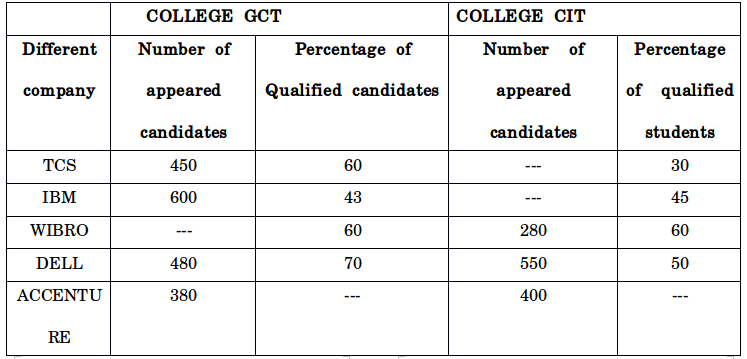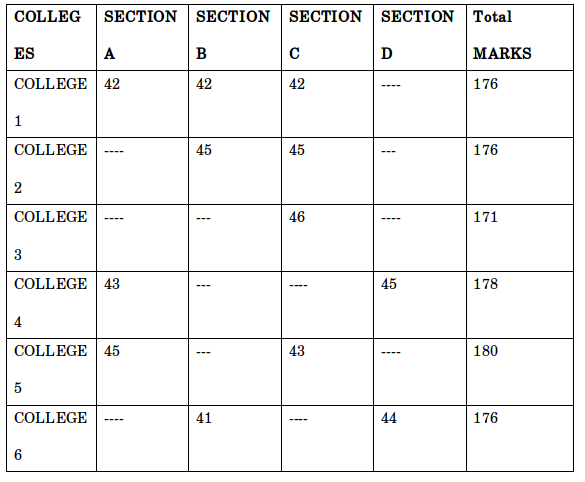## Important Data Interpretation For SBI PO : Set – 66

D.1-5) Study the following Table chart, and answer the following questions1) In GCT college, the ratio between the number of candidates qualified to DELL company and Accenture company is 14:9, what is the number of candidates qualified to Accenture company from the same college?

a) 252

b) 207

c) 216

d) 234

e) 198

c)

The ratio of the qualified candidates from the GCT College in DELL and Accenture Company the ratio is 14:9

Percentage of qualified Students in DELL= 480×70/100=336

Number of qualified students ratio is 14x=336

X=336/14=24

Number of candidates qualified from Accenture company is (9x) = 9×24=216

2) If the average number of candidates qualified in Wipro, Dell and Accenture Company from college CIT is 210. What is the number of candidates qualified in Accenture Company from the same College?

a) 191

b) 195

c) 183

d) 187

e) 179

d)

Average qualified Students from CIT College in Wipro, Dell and Accenture Company

=((280×60 )/100+(550×50 )/100+(400×x)/100)/3=210

168+175+4x=630

X=187

3) What is the difference between the number of candidates qualified to TCS company and IBM company from college GCT?

a) 12

b) 22

c) 14

d) 24

e) 16

a)

Required Difference=(450×60)/100-(600×43)/100=270-258=12

4) The number of appeared candidates from the CIT College for IBM Company is 100% more than for TCS Company. If the total number of qualified candidates from the college CIT in TCS and IBM together is 408, what is the number of appeared candidates from the college CIT for TCS Company?

a) 380

b) 360

c) 340

d) 320

e) 300

c)

The number of appeared candidates from CIT college in TCS company is x

The number of appeared candidate in IBM Company= x+(x×100)/100=2x

x×30/100+(2x×45)/100=408

3x/10+9x/10=408

12x=408×10

x=(408×10)/12=340

5) Out of the number of qualified candidates from GCT college in Wipro company, the ratio of male to female qualified candidates is 11:7.If the number of female qualified candidates from GCT college to Wipro is 126, what is the number of appeared candidates (Both male and female) from the college GCT for Wipro company?

a) 630

b) 510

c) 570

d) 690

e) 540

e)

Qualified students from GCT college in Wipro company the ratio between male and female is 11x: 7X

The number of qualified student Wipro (7x) =126 x=18

Qualified male member =18 ×11=198

Total number of qualified students=198+126=324

Total number of appeared students = y×60/100=342 (324×100)/60=540

D.6-10) Study the following information in the table. Answer the given question6) The ratio between the section D marks from college 1 and section C marks from college 4 is 5: 4. In college 4 section C mark is what percent of total marks of the same college

a) 56.17

b) 57.17

c) 56

d) 58.17

e) None of these

e)

In test D marks in College one= 176-42-42-42=50

Ratio between section D in college one : Section C in college Four

5x: 4x

In section D got (5x) =50

X=10

Section c mark=40

Percentage of marks section C in college four is =(100×40)/178=22.47

7) Find out the difference between the total sum of section C and section D marks from college 1 and the total sum of section B and section D marks from college 4

a) 4

b) 6

c) 3

d) 8

e) None of these

c)

Total marks in college one in section C and D is =42+50=92

Marks in Section B in college four= Total cut-off marks in college four- total marks in Section A+C+D

=178-43+50+45=50

Total marks in college four in section B and D =50+45=95

Required difference=95-92=3

8) In college 6 the ratio between section A and section C marks is 4:3.find the ratio between total sum of section C marks from college 2, college 3 and college 5 together and the total sum of section A and section B and section C marks from college 6?

a) 45:76

b) 67:66

c) 56:67

d) 45:78

e) None of these

b)

Ratio between marks in section A and section C in college six is: 4:3

Total marks in section A and C =total cut-off marks in college six-total marks in section B and D in college six= 176-41+44= 91

Ratio between their values is 52:39

Required ratio= 45+46+43: 41+39+44 => 134: 132 =>67:66

9) The ratio between the section C mark from college 2 and section B mark from college 5 is 9: 8. Find out the total sum of section D and section A marks from college 5?

a) 67

b) 56

c) 89

d) 97

e) None of these

d)

Total marks in section C in college 2 (9x) =45 x=45/9= 5

In college five section B marks is= 8×5=40

In college five section D marks is= total cut-off marks – total marks in section A ,B and C

=180-45+40+43=52

Required total =section D marks in college five +section A marks in college five

=52+45

=97

10) The total sum of section B and section C marks from college 2 is equals to total sum of section B and section C marks from college 3.In college 3,the ratio between the section A and section D marks is 2:1.find out the total sum of section A and section B marks from college 3?

a) 190

b) 45

c) 98

d) 78

e) None of these